## Suppose line segment XY has one endpoint at X(0,0). Question 1- Is (3,4) is the midpoint of line segment XY, what are the coordi

Question

Suppose line segment XY has one endpoint at X(0,0).

Question 1- Is (3,4) is the midpoint of line segment XY, what are the coordinates of point y?

Question 2- What are the coordinates of Y if (3,4) is 1/3 of the way from X to Y?

in progress 0
4 months 2021-09-05T12:40:51+00:00 1 Answers 0 views 0

## Answers ( )

1) The coordinates of point Y are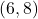, 2) The coordinates of point Y are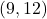.

Step-by-step explanation:

1) We know that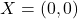and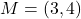, being the latter one the midpoint of line segment XY. Vectorially, the location of point Y is determined by the following expression: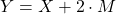Now we solve the expression above: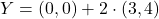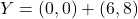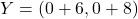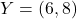The coordinates of point Y are.

2) We know thatand, being the latter one a third of the way of line segment XY.

Vectorially, the location of point Y is determined by the following expression: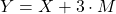Now we solve the expression above: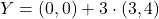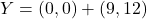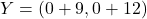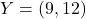The coordinates of point Y are.